# Being Asked Opinion by Recall Network

Network of members that ask for an opinion by recall

## Examples

### Basic Examples

Retrieve the graph:

 In:=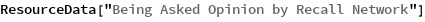Out=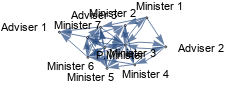Summary properties:

 In:=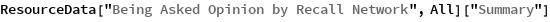Out=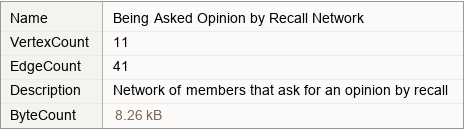### Basic Applications

Show the opinions of members asked by others:

 In:=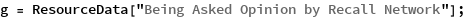In:=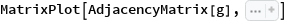Out=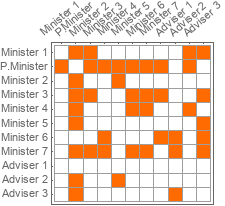Wolfram Research, "Being Asked Opinion by Recall Network" from the Wolfram Data Repository (2019)